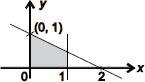# User Forum

Subject :IMO    Class : Class 9

In the rectangular coordinate system given below, the shaded region is bounded by two straight lines. Which of the following is not an equation of one of the boundary lines?A x = 0
B x = 1
C xy = 0
D x + 2y = 2